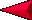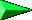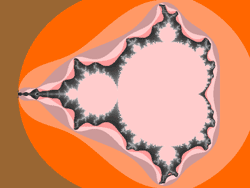Back EQUATIONS OF SCIENCELesson 1 - Page 5 NextUsed with permission. Copyright 1996, James P. Crutchfield.

Chaos theory tries to make sense of apparently disordered systems.  An early pioneer in chaos theory was  Edward Lorenz (1917- ).  In 1960 he was doing meteorological research trying to develop models for predicting weather.  His formulas started to reveal random patterns which interested him.  So he started to work on formulas that analyzed unpredictable behavior.   He developed the idea that the flapping of a butterfly's wing will create a chaotic disturbance in the atmosphere called the butterfly effect (click on picture for animation).  A small disturbance can cause a large scale atmospheric motion, so that the long term behavior becomes impossible to forecast. Scientists in other fields of science started to see this behavior in other systems.Details of the Koch snowflake

Helge von Koch (1870-1924), a mathematician, captured a similar  idea earlier.  He created one of the first fractal images now called the Koch snowflake or star.  Imagine an equilateral triangle then keep adding equilateral triangles that fit.  A pattern starts to emerge on this self-duplicating pattern.  If you keep doing this the line around the figure keeps getting larger and larger.  Mathematicians invented fractal dimensions or fractals.  Later a scientist, Mitchell Fergenbaum (1944-  ) looked at this growth and noted that they grow at a constant rate of 4.669.  The number kept coming up when he was comparing systems.   This was a revolutionary discovery.  Mathematical systems that seemed chaotic were not really.  The power of numbers can now make sense out of a chaotic system!

When you plot fractal equations you graphical derive fractal images.  For example the Mandelbrot set (equations z = z2 + c) creates a wonderful image.  Applications of fractal images are abundant from chaotic heart beats to charting coastlines as well as computer art to music.Keep adding equilateral trianglesMandelbrot image